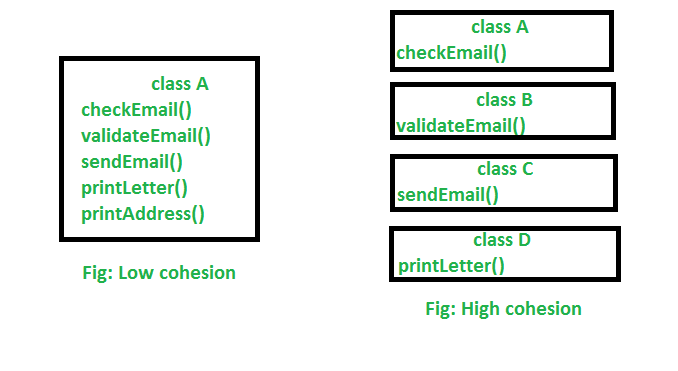GFG App
Open AppBrowser
Continue

# Cohesion in Java

Cohesion in Java is the Object-Oriented principle most closely associated with making sure that a class is designed with a single, well-focused purpose. In object-oriented design, cohesion refers to how a single class is designed.

Note: The more focused a class is, the more is the cohesiveness of that class.

The advantage of high cohesion is that such classes are much easier to maintain (and less frequently changed) than classes with low cohesion. Another benefit of high cohesion is that classes with a well-focused purpose tend to be more reusable than other classes.

Example: Suppose we have a class that multiplies two numbers, but the same class creates a pop-up window displaying the result. This is an example of a low cohesive class because the window and the multiplication operation don’t have much in common. To make it high cohesive, we would have to create a class Display and a class Multiply. The Display will call Multiply’s method to get the result and display it. This way to develop a high cohesive solution.

Let us understand the structure of the high cohesive program:

## Java

 `// Java program to illustrate` `// high cohesive behavior`   `class` `Multiply {` `  `  `    ``int` `a = ``5``;` `    ``int` `b = ``5``;` `  `  `    ``public` `int` `mul(``int` `a, ``int` `b)` `    ``{` `        ``this``.a = a;` `        ``this``.b = b;` `        ``return` `a * b;` `    ``}` `} `   `class` `Display {` `    ``public` `static` `void` `main(String[] args)` `    ``{` `        ``Multiply m = ``new` `Multiply();` `        ``System.out.println(m.mul(``5``, ``5``));` `    ``}` `}`

Output

`25`

## Java

 `// Java program to illustrate` `// high cohesive behavior`   `class` `Name {` `    ``String name;` `    ``public` `String getName(String name)` `    ``{` `        ``this``.name = name;` `        ``return` `name;` `    ``}` `} `   `class` `Age {` `    ``int` `age;` `    ``public` `int` `getAge(``int` `age)` `    ``{` `        ``this``.age = age;` `        ``return` `age;` `    ``}` `} `   `class` `Number {` `    ``int` `mobileno;` `    ``public` `int` `getNumber(``int` `mobileno)` `    ``{` `        ``this``.mobileno = mobileno;` `        ``return` `mobileno;` `    ``}` `} `   `class` `Display {` `    ``public` `static` `void` `main(String[] args)` `    ``{` `        ``Name n = ``new` `Name();` `        ``System.out.println(n.getName(``"Geeksforgeeks"``));` `        ``Age a = ``new` `Age();` `        ``System.out.println(a.getAge(``10``));` `        ``Number no = ``new` `Number();` `        ``System.out.println(no.getNumber(``1234567891``));` `    ``}` `}`

Output

```Geeksforgeeks
10
1234567891```

Pictorial view of high cohesion and low cohesion:Explanation: In the above image, we can see that in low cohesion only one class is responsible to execute lots of jobs that are not in common which reduces the chance of reusability and maintenance. But in high cohesion, there is a separate class for all the jobs to execute a specific job, which results in better usability and maintenance.

Difference between high cohesion and low cohesion:

• High cohesion is when you have a class that does a well-defined job. Low cohesion is when a class does a lot of jobs that don’t have much in common.
• High cohesion gives us better-maintaining facility and Low cohesion results in monolithic classes that are difficult to maintain, understand and reduce re-usability